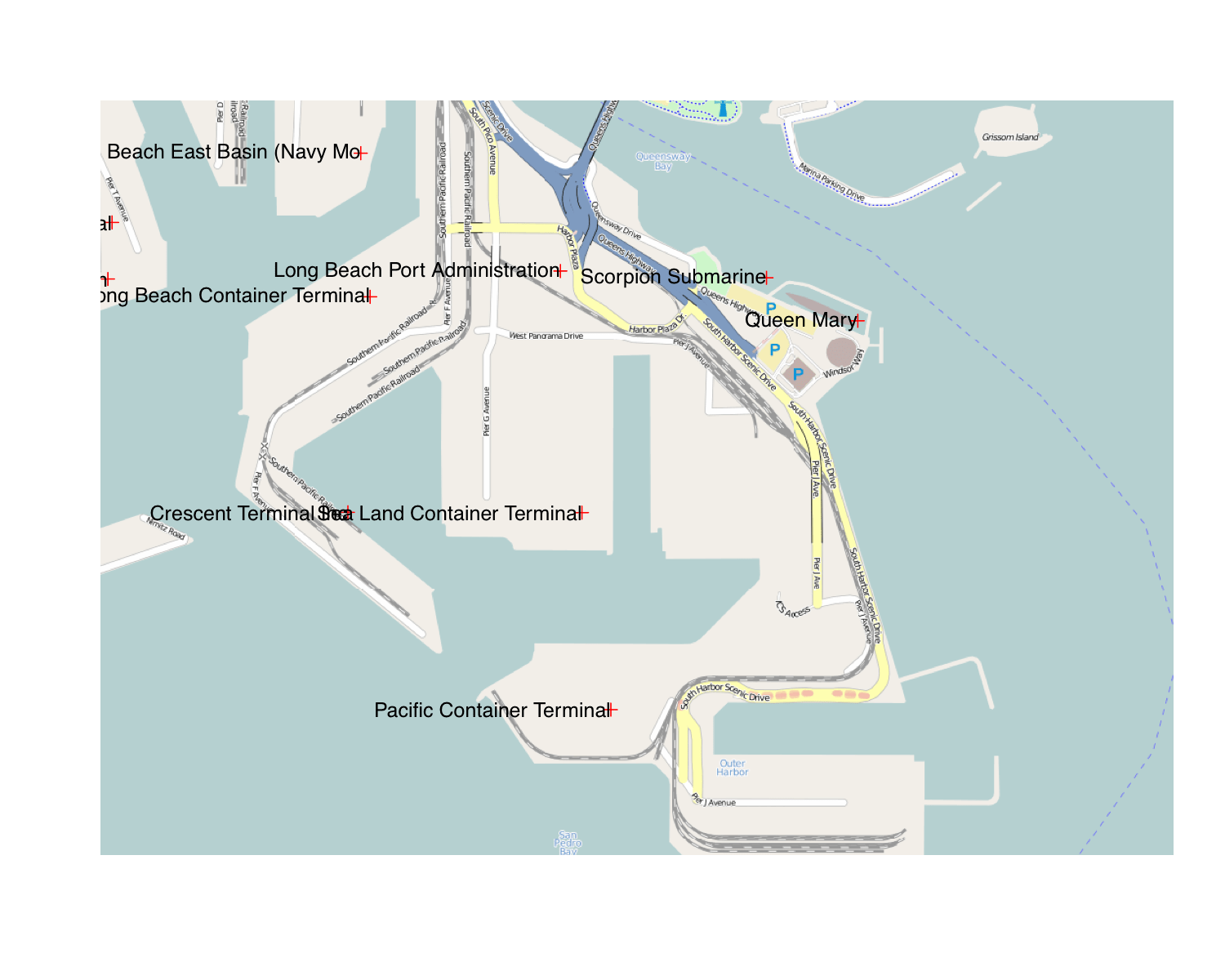Want to share your content on R-bloggers? click here if you have a blog, or here if you don't.

A new package OpenStreetMap has been released to CRAN this week which is designed to allow you to easily add satellite imagery, or open street maps to your plots. Raster maps are a great way to add context to your spatial data with a minimum outlay of effort.

The syntax in OpenStreetMap is fairly simple, just give it a bounding box in lat/long and it will download a high quality raster image ready for plotting

` library(OpenStreetMap) library(rgdal) map <- openmap(c(70,-179), c(-70,179)) plot(map)`(click for higher quality image)

The above code downloads multiple map tiles and stitches together, with the level of zoom determined automatically. Unlike RGoogleMaps no files are created or stored on the hard drive. The plot gets is images from the mapnik server, which can provide street level information. In my opinion, there rendering looks pretty clean as well.

We can also access satellite imagery though Bing.

` map <- openmap(c(70,-179), c(-70,179),type='bing') plot(map)`Now, that is all fine and dandy, but kind of useless unless you are able to combine it with your own data. Open street map, and Bing (and Google maps) use a particular form of the Mercator projection which has the properties that angles are preserved, and tiles on multiple zoom levels can be stitched together. You can access this projection with the ‘osm’ function.

In terms of combining maps with your data there are two options. The data can be transformed to the osm() projection, or the raster map can be translated to whatever projection the data are in. Here is an example of the first option:

`library(UScensus2000) data(california.tract) lat <- c(43.834526782236814,30.334953881988564) lon <- c(-131.0888671875 ,-107.8857421875) southwest <- openmap(c(lat,lon),c(lat,lon),zoom=6,'osm') california.tract <- spTransform(california.tract,osm()) plot(southwest) plot(california.tract,add=TRUE,col=([email protected]\$med.age>40)+4) `

Here we take a map from the UScensus2000 package, transform it to mercator coordinates, and make a choropleth. The spTransform function is a great easy way to project your data into different map coordinate systems.We may also want to go the other way and transform the image. The openproj function can transform open street maps to different projections. Here is an example combining OpenStreetMap with the maps library by projecting the map into longlat coordinates.

`map <- openmap(c(70,-179), c(-70,179),type='bing') map_longlat <- openproj(map, projection = "+proj=longlat") plot(map_longlat,raster=TRUE) map("world",col="red",add=TRUE)`but, we are not just limited to the longlat projection, we can also do weirder ones like the lambert conic conformal.

`map <- openmap(c(70,-179), c(40,179),zoom=2,type='bing') map_longlat <- openproj(map) #Lambert Conic Conformal (takes some time...) map_llc <- openproj(map_longlat, projection= "+proj=lcc +lat_1=33 +lat_2=45 +lat_0=39 +lon_0=-96") plot(map_llc,raster=TRUE) #add choropleth data(states) st_llc <- spTransform(states,CRS("+proj=lcc +lat_1=33 +lat_2=45 +lat_0=39 +lon_0=-96")) plot(st_llc,add=T,col=heat.colors(48,.4)[slot(st_llc,"data")[["ORDER_ADM"]]]) `Now, I have no idea why you would want to use this projection, but it is pretty cool none the less.

One of the best uses for raster maps is in street level data, where it is particularly important to give the reader contextual information. Here is a plot of some locations in the Long Beach harbor, using the LA_places dataset.

`data(LA_places) xy <- coordinates(LA_places) map <- openmap(c(33.760525217369974,-118.22052955627441), c(33.73290566922855,-118.17521095275879)) png(width = 1000, height = 1000) plot(map,raster=TRUE) plot(LA_places,add=TRUE,col="red") text(xy[,1],xy[,2],slot(LA_places,"data")[,'NAME'],adj=1)`If you are a Deducer user, the DeducerSpatial package provides a GUI for spatial plotting using the OpenStreetMap package.

====================

A note from R-bloggers editor:

If you get –
Error: ‘merge’ is not an exported object from ‘namespace:raster’

The fix is to install the raster package from here:
install.packages(“raster”, repos=”http://R-Forge.R-project.org”)

Bug report was kindly submitted by “Jos”.